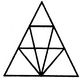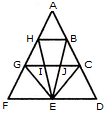# Non Verbal Reasoning - Analytical Reasoning - Discussion

Discussion Forum : Analytical Reasoning - Section 1 (Q.No. 4)
4.

Find the number of triangles in the given figure.12
18
22
26
Explanation:

The figure may be labelled as shown.The simplest triangles are AHB, GHI, BJC, GFE, GIE, IJE, CEJ and CDE i.e. 8 in number.

The triangles composed of two components each are HEG, BEC, HBE, JGE and ICE i.e. 5 in number.

The triangles composed of three components each are FHE, GCE and BED i.e. 3 in number.

There is only one triangle i.e. AGC composed of four components.

There is only one triangle i.e. AFD composed of nine components.

Thus, there are 8 + 5 + 3 + 1 + 1 = 18 triangles in the given figure.

Discussion:
32 comments Page 1 of 4.

JIYA said:   3 years ago
Please anyone tell me the correct way to solve this.

Rit said:   4 years ago
Total no nodes (8) + 2 * (5) inner nodes (h, b, g, c, e) = 18.

Sharad Bhatta said:   5 years ago
I don't understand the concept of the triangle components.

Please, anybody, give me some ideas.

Ritika said:   5 years ago
I don't think so, that two components forming with 4 letters as HBE &BEC both are two components triangle but HBE made with 5 letters and BEC made with 4 letters.

Akter ali said:   5 years ago
The simple triangle means forming with 3 letters.

Two components forming with 4 letters.
Three components forming with 5 letters.

Mansi Sahu said:   6 years ago
I don't understand the proper meaning of components, please anybody can explain me clearly?

Anonymus said:   6 years ago
The simplest triangles are AHB, GHI, BJC, GFE, GIE, IJE, CEJ and CDE which equals 8 in number.
The triangles composed of two components each are HEG, BEC, HBE, JGE and ICE = 5 in number.

The triangles composed of three components each are FHE, GCE and BED which are 3 in number.

There is only one triangle i.e. AGC composed of four components.

There is only one triangle i.e. AFD composed of nine components(sides of triangles.

Thus, there are 8 + 5 + 3 + 1 + 1 = 18 triangles in the given figure.

Himanshu tomar said:   7 years ago
Is there any short trick to find the answer?
(2)

Pratham Verma said:   7 years ago
Count by nodes 2Rupesh.

Rupesh singh said:   7 years ago
Is there any other way to solve it easily.